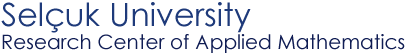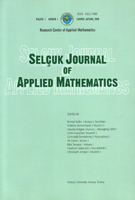Selþuk Journal of Applied Mathematics www.selcuk.edu.trSelþuk Journal of
Applied Mathematics

Summer-Autumn, 2002
Volume  3
Number 2

Research Center of
Applied Mathematics

 SJAM Summer-Autumn 2002, Volume 3 - Number 2 Optimal systems of symmetry subalgebras for big models of gasdynamics Salavat Khabirov Institute of Mechanics, UB RAS , Marx street 12, 450000 Ufa, Russia    email : habirov@anrb.ru ;    Received: March 26, 2001 Summary For an arbitrary state equation the gasdynamics equations admit the 11-dimensional Lie algebra of transformations. But there are 13 special state equations for which the corresponding Lie algebra of transformations may be expanded. In this event we call the gasdynamics equations the big models. Some of the Lie algebras under consideration are similar to one another and the algebra structure may be different under further specialization of the state equation. It was noted that only two specializations of the state equation need to be added into consideration. In the paper we make a review of extensions for which the optimal systems of subalgebras may be found and calculate these optimal systems. Each of the extensions is presented as the semidirect sum of the Abelian 6-dimensional ideal and a subalgebra. The normalized optimal systems of these subalgebras are calculated. Key words gasdynamics, state equation, Lie algebra of transformations, optimal systems of subalgebras. 2000 Mathematics Subject Classification : 37K30, 76N15 Article in PS format (235 Kb) Article in ZIP format (87.8 Kb)
 The article is available to read in  Ghostview format
 [Home] [contact]